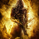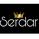145 views
When I look at the analysis, I am bullish because of some reason. Let's explain,

1) Risk/Reward Ratio is 1.9, so close the 2. For me, the ratio is more than 2 or close 2, this means the candles are going down.
2) According to Fibonacci Levels, the next stop is 0.0008850, and then the resistance line is 0.0010966.
3)EngineeringRobo's signals are positive.

Therefore, I would prefer to buy it.
Start your FREE trial with Engineering Robo: